## Write the equation of the line passing through points ( -2,-5) and (1,1)

Question

Write the equation of the line passing through points ( -2,-5) and (1,1)

in progress 0
7 months 2021-08-01T16:29:34+00:00 1 Answers 4 views 0

The equation of the line is:Step-by-step explanation:

Given the points

• (-2, -5)
• (1, 1)

Finding the slope between the points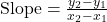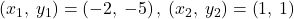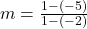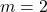We know the slope-intercept form of the line equation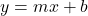where m is the slope and b is the slope-intercept form

substituting the value m=2 and the point (-2, -5) to find the b-intercept-5 = 2(-2) + b

b = -5+4

b = -1

Now, substituting m=2 and b=-1 in the slope-intercept form to get the equation of a line

y=mx+b

y=2x+(-1)

y=2x-1

Thus, the equation of the line is: